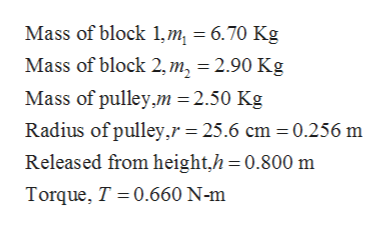# Two blocks are connected by massless string that is wrapped around a pulley. Block 1 has a mass m1=6.70m1=6.70 kg, block 2 has a mass m2=2.90m2=2.90 kg, while the pulley has a mass of 2.50 kg and a radius of 25.6 cm. When the pulley turns, there is friction in the axel that exerts a torque of magnitude 0.660 N m.If block 1 is released from rest at a height h=0.800h=0.800 m, how long does it take to drop to the floor?

Question
171 views

Two blocks are connected by massless string that is wrapped around a pulley. Block 1 has a mass m1=6.70m1=6.70 kg, block 2 has a mass m2=2.90m2=2.90 kg, while the pulley has a mass of 2.50 kg and a radius of 25.6 cm. When the pulley turns, there is friction in the axel that exerts a torque of magnitude 0.660 N m.

If block 1 is released from rest at a height h=0.800h=0.800 m, how long does it take to drop to the floor?

check_circle

Step 1

Given,help_outlineImage TranscriptioncloseMass of block 1,m, 6.70 Kg Mass of block 2, m, = 2.90 Kg Mass of pulley,m = 2.50 Kg Radius of pulley,r = 25.6 cm 0.256 m Released from height,h 0.800 m Torque, T 0.660 N-m fullscreen
Step 2

Tension and weights acting on the block are shown below,

Step 3

As friction is acting about pulley so different amount of tension will be acting at both sides of pulley. This difference in tension in str...

### Want to see the full answer?

See Solution

#### Want to see this answer and more?

Solutions are written by subject experts who are available 24/7. Questions are typically answered within 1 hour.*

See Solution
*Response times may vary by subject and question.
Tagged in

### Physics